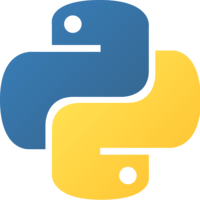This lesson is for members. Join us? Get access to all 3,000+ tutorials + a community with expert developers around the world.

Unlock This Lesson
1×
Become a member
to unlock all featuresLevel Up!

Access all courses & lessons on egghead today and lock-in your price for life.

Autoplay

Create a Dense Neural Network for Multi Category Classification with Keraspython
^3.0.0

We’ll take a network set up for binary classification, and turn it into a network that can take 3 or more classes. This network will let us go beyond classifying data into only two categories, and will allow us to expand to any number of categories (for example: “dog” vs “cat” vs “mouse”). Then, we’ll use real data from a csv to train and test that network.CodeYou must be a Member to view code

Access all courses and lessons, track your progress, gain confidence and expertise.

Become a Member
and unlock code for this lessonDiscussTranscript

Instructor: We're training the neural networks on a CSV with two classes, zero for low and one for high. Now, we're going to switch that to a new dataset. This is the Iris dataset, which is a common training set used to test neural networks. Each row represents a different flower and each flower has four data points, the Sepal Length, Sepal Width, Petal Length, and Petal Width.

The final column is the class of the flower, which is a zero, a one, or two. A zero represents Iris setosa, a one is Iris versicolor, and two is Iris virginica. We can import the iris.csv file. We have three classes, but before we just had two. Now, we have to convert the network from a binary classification network to a multi-class classification network.

First, we can't use binary cross entropy anymore, because that's only for two-class problems. We'll update that to categorical cross entropy, which can use any number of classes. Categorical cross entropy, however, can't take up the flower classes as just a number like zero, one, or two, but instead, it needs to have the class represented by a one-hot encoded vector.

Keras has a built-in function to do that translation for us. Import to_categorical from keras.utils.np_utils. If we check the Y values we have now, we have an array filled with zero's, one's, and two's. We can call to_categorical and pass in our Y values.

Now, we have an array of one-hot encoded vectors, which means the index zero value is a one, if the class was a zero, the index one value is a one, if the class was a one, and the index two value is a one, if the class was a two.

Since we are using validation split in the model fit and we have auto-data, we also want to make sure to shuffle the data before we do a fit, whereas the validation set would only select from the end of the file, which would only ever include the flowers of class two.

We have the data in the correct format for the fit network call now. Our network output has to change from a single zero or one value to a one-hot encoded vector of length three. First, we have to change the size of the output from one to three, which means, we want to reconsider our sigmoid activation function.

We can think of the three outputs as the probability that the inputs are each one of the classes. In this example output, there is a 10 percent chance that the flower is Iris setosa, a 20 percent chance that the flower is Iris versicolor, and a 70 percent chance the flower is Iris virginica.

By using a sigmoid, means we can range from zero to one for all of the probabilities. What if we get an output where all the probabilities are 09. To avoid this type of case, we'll use an activation function called Softmax, which will ensure that all three probabilities add to one, which will help our neural network decide which class it might belong to then we can run our model.

In only one hundred epox, we're getting fairly good results with our new multi-class model.